Internal angles

The ABCD is an isosceles trapezoid, which holds:
|AB| = 2 |BC| = 2 |CD| = 2 |DA|:

On its side BC is a K point such that |BK| = 2 |KC|, on its side CD is the point L such that |CL| = 2 |LD|, and on its side DA the point M is such that | DM | = 2 |MA|. Determine the internal angles of the KLM triangle.

Result

∠KLM =  120 °
∠LMK =  30 °
∠MKL =  30 °

Leave us a comment of example and its solution (i.e. if it is still somewhat unclear...):

Showing 1 comment:Math student
Help. First, look at the inner angles of the ABCD trapezoid.

Solution. It follows from the assumptions that the center line of the AB segment with the vertices C and D divides the ABCD trapezoid into three identical equilateral triangles. Therefore, the magnitude of internal angles in the trapezoid at A and B vertices is equal to 60 °
And at the C and D vertices 120 °. It follows from the specification that the triangles LCK and MDL are the same (according to the sentence above). Therefore, both the KL and LM lines and the designated pairs of angles are the same; The magnitudes of these angles are denoted α and β. The triangle KLM is isosceles and the angles at the base are the same; Their size is denoted by δ and the size of the angle KLM is denoted by γ.

From the sum of the inner angles in the KCL triangle we derive
α + β = 180° − 120° = 60°

The sum of the three marked angles with the vertex L is a straight angle, therefore
γ = 180° − (α + β) = 120°
Finally, we deduce the sum of inner angles in the triangle KLM
δ = (180° − 120°)/2 = 30°

The internal angles of the triangle KLM are 30° and 120°Next similar examples:

1. Trapezium internal anglesA trapezium where AB is parallel to CD, has angle A : angle D = 4 :5, angle B = 3x-15 and angle C = 4x+20. Find angle A, B, C and D.
2. Internal anglesFind the internal angles of the triangle ABC if the angle at the vertex C is twice the angle at the B and the angle at the vertex B is 4 degrees smaller than the angle at the vertex A.
3. Isosceles trapezoid v3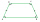In an isosceles trapezoid ABCD is the size of the angle β = 81° Determine size of angles α, γ and δ.
4. Angles in triangleThe triangle is ratio of the angles β:γ = 6:8. Angle α is 40° greater than β. What are the size of angles of the triangle?
5. AnglesIn the triangle ABC, the ratio of angles is: a:b = 4: 5. The angle c is 36°. How big are the angles a, b?
6. Triangle 42Triangle BCA. Angles A=119° B=(3y+14) C=4y. What is measure of triangle BCA=?
7. A kite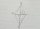ABCD is a kite. Angle OBC = 20° and angle OCD = 35°. O is the intersection of diagonals. Find angle ABC, angle ADC and angle BAD.
8. Trapezium ABCDIn the figure, ABDC is a trapezium in which AB || CD. line segments RN and LM are drawn parallel to AB such that AJ=JK=KP. If AB=0.5m and AP=BQ=1.8m, find the lengths of AC, BD, RN and LM. angle D=angle C=60
9. Trapezoid - RRFind the area of the right angled trapezoid ABCD with the right angle at the A vertex; a = 3 dm b = 5 dm c = 6 dm d = 4 dm
10. Isosceles trapezium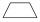Trapezoid YSED (YS||ED) is isosceles. The size of the angle at vertex Y is 17 degrees. Calculate the size of the angle at vertex E.
11. KLMNIn the trapezoid KLMN is given this informations: 1. segments KL and MN are parallel 2. segments KL and KM has same length 3. segments KN, NM and ML has same length. Determine the size of the angle KMN.
12. Reference angleFind the reference angle of each angle: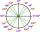What is greater angle? -0.7 radians or -0.7π radians?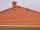Tiles are stacked in rows on the trapezoidal shaped roof. At the ridge is 15 tiles and each subsequent row has one more tile than in the previous row. How many tiled is covered roof if lowermost row has 37 tiles?Cat's school use a special numerical axis. The distance between the numbers 1 and 2 is 1 cm, the distance between the numbers 2 and 3 is 3 cm, between the numbers 3 and 4 is 5 cm and so on, the distance between the next pair of natural numbers is always inIt is true that the middle traverse bisects the triangle?We want to prove the sentence: If the natural number n is divisible by six, then n is divisible by three. From what assumption we started?